# Coordinate Geometry Exercise 7.2

Coordinate Geometry Exercise 7.2

1. Find the coordinates of the point which divides the join of (- 1, 7) and (4, - 3) in the ratio 2:3.

Let P(xy) be the required point. Using the section formula, we get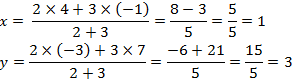Therefore, the point is (1, 3).

2. Find the coordinates of the points of trisection of the line segment joining (4, -1) and (-2, -3).Let P (x1y1) and Q (x2y2) are the points of trisection of the line segment joining the given points i.e., AP = PQ = QB

Therefore, point P divides AB internally in the ratio 1:2.3. To conduct Sports Day activities, in your rectangular shaped school ground ABCD, lines have been drawn with chalk powder at a distance of 1 m each. 100 flower pots have been placed at a distance of 1 m from each other along AD, as shown in the following figure. Niharika runs 1/4th the distance AD on the 2nd line and posts a green flag. Preet runs 1/5th the distance AD on the eighth line and posts a red flag. What is the distance between both the flags? If Rashmi has to post a blue flagexactly halfway between the line segment joining the two flags, where should she post her flag?It can be observed that Niharika posted the green flag at 1/4th of the distance AD i.e., (1×100/4)m = 25m from the starting point of 2nd line. Therefore, the coordinates of this point G is (2, 25).
Similarly, Preet posted red flag at 1/5 of the distance AD i.e., (1×100/5) m = 20m from the starting point of 8th line. Therefore, the coordinates of this point R are (8, 20).
Distance between these flags by using distance formula = GRTherefore, Rashmi should post her blue flag at 22.5m on 5th line.

4. Find the ratio in which the line segment joining the points (-3, 10) and (6, - 8) is divided by (-1, 6).

Let the ratio in which the line segment joining ( -3, 10) and (6, -8) is divided by point ( -1, 6) be k : 1.Therefore, -1 = 6k-3/k+1
-k - 1 = 6k -3
7k = 2
k = 2/7
Therefore, the required ratio is 2:7.

5. Find the ratio in which the line segment joining A (1, - 5) and B (- 4, 5) is divided by the x-axis. Also find the coordinates of the point of division.

Let the ratio in which the line segment joining A (1, - 5) and B ( - 4, 5) is divided by x-axisbe.
Therefore, the coordinates of the point of division is (-4k+1/k+1, 5k-5/k+1).

We know that y-coordinate of any point on x-axis is 0.

∴ 5k-5/k+1 = 0

Therefore, x-axis divides it in the ratio 1:1.6. If (1, 2), (4, y), (x, 6) and (3, 5) are the vertices of a parallelogram taken in order, find and y.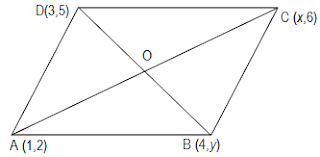Let A,B,C and D be the points (1,2) (4,y), (x,6) and (3,5) respectively.

Mid point of diagonal AC isand Mid point of Diagonal BD isSince the diagonals of a parallelogram bisect each other, the mid point of AC and BD are same.

∴ x+1/2 = 7/2 and 4 = 5+y/2

⇒ x + 1 = 7 and 5 + y = 8

⇒ x = 6 and y = 3

7. Find the coordinates of a point A, where AB is the diameter of circle whose centre is (2, - 3) and B is (1, 4).

Let the coordinates of point A be (xy).
Mid-point of AB is (2, - 3), which is the center of the circle.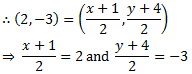⇒ x + 1 = 4 and y + 4 = -6

⇒ x = 3 and y = -10
Therefore, the coordinates of A are (3,-10).

8. If A and B are (–2, –2) and (2, –4), respectively, find the coordinates of P such that AP = 3/7 AB and P lies on the line segment AB.The coordinates of point A and B are (-2,-2) and (2,-4) respectively.

Since AP = 3/7 AB

Therefore, AP:PB = 3:4

Point P divides the line segment AB in the ratio 3:4.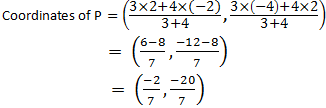9. Find the coordinates of the points which divide the line segment joining A (- 2, 2) and B (2, 8) into four equal parts.From the figure, it can be observed that points X, Y, Z are dividing the line segment in a ratio 1:3, 1:1, 3:1 respectively.10. Find the area of a rhombus if its vertices are (3, 0), (4, 5), (-1, 4) and (-2,-1) taken in order. [Hint: Area of a rhombus = 1/2(product of its diagonals)]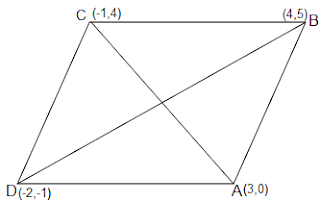Let (3, 0), (4, 5), ( - 1, 4) and ( - 2, - 1) are the vertices A, B, C, D of a rhombus ABCD.## TS PRC2015

Teacher ModulesSSC MODEL PAPERSMATHS WORK SHEETSImp web linksSENIORITY LISTSREPRESENTATIONSHEALTH TIPSRTI ACTTSWRTU